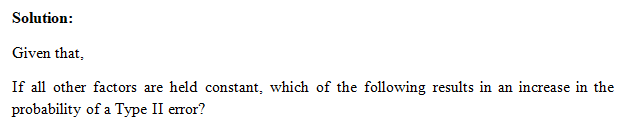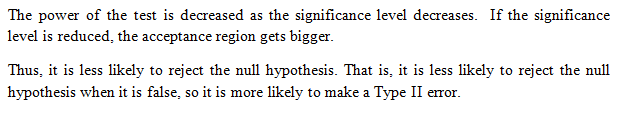# If all other factors are held constant, which of the following results in an increase in the probability of a Type IIII error?  The true parameter is farther from the value of the null hypothesis.AThe sample size is increased.BThe significance level is decreased.CThe standard error is decreased.DThe probability of a Type IIII error cannot be increased, only decreased.

Question
86 views

If all other factors are held constant, which of the following results in an increase in the probability of a Type IIII error?

• The true parameter is farther from the value of the null hypothesis.

A
• The sample size is increased.

B
• The significance level is decreased.

C
• The standard error is decreased.

D
• The probability of a Type IIII error cannot be increased, only decreased.

check_circle

Step 1Step 2...

### Want to see the full answer?

See Solution

#### Want to see this answer and more?

Solutions are written by subject experts who are available 24/7. Questions are typically answered within 1 hour.*

See Solution
*Response times may vary by subject and question.
Tagged in